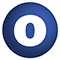geotrellis

# OpX

#### object OpX

Linear Supertypes
AnyRef, Any
Ordering
1. Alphabetic
2. By inheritance
Inherited
1. OpX
2. AnyRef
3. Any
1. Hide All
2. Show all
Learn more about member selection
Visibility
1. Public
2. All

### Type Members

1. #### type DI = DummyImplicit

Add simple syntax for creating an operation.

Add simple syntax for creating an operation.

Define a function after op function that returns:

1) A literal value, e.g. val PlusOne = op { (i:Int) => i + 1 }

2) An operation to be executed: val LocalPlusOne ( (r:Raster, i:Int) => local.Add(r,i + 1) )

3) Or a StepResult (which indicates success or failure) val PlusOne = op { (i:Int) => Result(i + 1) }

### Value Members

1. #### final def !=(arg0: AnyRef): Boolean

Definition Classes
AnyRef
2. #### final def !=(arg0: Any): Boolean

Definition Classes
Any
3. #### final def ##(): Int

Definition Classes
AnyRef → Any
4. #### final def ==(arg0: AnyRef): Boolean

Definition Classes
AnyRef
5. #### final def ==(arg0: Any): Boolean

Definition Classes
Any
6. #### final def asInstanceOf[T0]: T0

Definition Classes
Any
7. #### def clone(): AnyRef

Attributes
protected[java.lang]
Definition Classes
AnyRef
Annotations
@throws()
8. #### final def eq(arg0: AnyRef): Boolean

Definition Classes
AnyRef
9. #### def equals(arg0: Any): Boolean

Definition Classes
AnyRef → Any
10. #### def finalize(): Unit

Attributes
protected[java.lang]
Definition Classes
AnyRef
Annotations
@throws()
11. #### final def getClass(): Class[_]

Definition Classes
AnyRef → Any
12. #### def hashCode(): Int

Definition Classes
AnyRef → Any
13. #### final def isInstanceOf[T0]: Boolean

Definition Classes
Any
14. #### final def ne(arg0: AnyRef): Boolean

Definition Classes
AnyRef
15. #### final def notify(): Unit

Definition Classes
AnyRef
16. #### final def notifyAll(): Unit

Definition Classes
AnyRef
17. #### def op[A, B, C, D, T](f: (A, B, C, D) ⇒ T)(implicit m: Manifest[T], n: DI, o: DI): (Op[A], Op[B], Op[C], Op[D]) ⇒ Op4[A, B, C, D, T]

Create an operation from a 4-arg function that returns a literal value.

18. #### def op[A, B, C, D, T](f: (A, B, C, D) ⇒ Op[T])(implicit m: Manifest[T], n: DI): (Op[A], Op[B], Op[C], Op[D]) ⇒ Op4[A, B, C, D, T]

Create an operation from a 4-arg function that returns an operation.

19. #### def op[A, B, C, D, T](f: (A, B, C, D) ⇒ StepOutput[T])(implicit m: Manifest[T]): (Op[A], Op[B], Op[C], Op[D]) ⇒ Op4[A, B, C, D, T]

Create an operation from a 4-arg function that returns StepOutput.

20. #### def op[A, B, C, T](f: (A, B, C) ⇒ T)(implicit m: Manifest[T], n: DI, o: DI): (Op[A], Op[B], Op[C]) ⇒ Op3[A, B, C, T]

Create an operation from a 3-arg function that returns a literal value.

21. #### def op[A, B, C, T](f: (A, B, C) ⇒ Op[T])(implicit m: Manifest[T], n: DI): (Op[A], Op[B], Op[C]) ⇒ Op3[A, B, C, T]

Create an operation from a 3-arg function that returns an operation.

22. #### def op[A, B, C, T](f: (A, B, C) ⇒ StepOutput[T])(implicit m: Manifest[T]): (Op[A], Op[B], Op[C]) ⇒ Op3[A, B, C, T]

Create an operation from a 3-arg function that returns StepOutput.

23. #### def op[A, B, T](f: (A, B) ⇒ T)(implicit m: Manifest[T], n: DI, o: DI): (Op[A], Op[B]) ⇒ Op2[A, B, T]

Create an operation from a 2-arg function that returns a literal value.

24. #### def op[A, B, T](f: (A, B) ⇒ Op[T])(implicit m: Manifest[T], n: DI): (Op[A], Op[B]) ⇒ Op2[A, B, T]

Create an operation from a 2-arg function that returns an operation.

25. #### def op[A, B, T](f: (A, B) ⇒ StepOutput[T])(implicit m: Manifest[T]): (Op[A], Op[B]) ⇒ Op2[A, B, T]

Create an operation from a 2-arg function that returns StepOutput.

26. #### def op[A, T](f: (A) ⇒ T)(implicit m: Manifest[T], n: DI, o: DI): (Op[A]) ⇒ Op1[A, T]

Create an operation from a 1-arg function that returns a literal value.

Create an operation from a 1-arg function that returns a literal value.

For example:

val PlusOne = op { (i:Int) => i + 1 }

27. #### def op[A, T](f: (A) ⇒ Op[T])(implicit m: Manifest[T], n: DI): (Op[A]) ⇒ Op1[A, T]

Create an operation from a 1-arg function that returns an operation to be executed.

Create an operation from a 1-arg function that returns an operation to be executed.

For example:

val LocalPlusOne ( (r:Raster, i:Int) => local.Add(r,i + 1) )

28. #### def op[A, T](f: (A) ⇒ StepOutput[T])(implicit m: Manifest[T]): (Op[A]) ⇒ Op1[A, T]

Create an operation from a 1-arg function that returns StepOutput.

Create an operation from a 1-arg function that returns StepOutput.

For example:

val PlusOne = op { (i:Int) => Result(i + 1) }

29. #### final def synchronized[T0](arg0: ⇒ T0): T0

Definition Classes
AnyRef
30. #### def toString(): String

Definition Classes
AnyRef → Any
31. #### final def wait(): Unit

Definition Classes
AnyRef
Annotations
@throws()
32. #### final def wait(arg0: Long, arg1: Int): Unit

Definition Classes
AnyRef
Annotations
@throws()
33. #### final def wait(arg0: Long): Unit

Definition Classes
AnyRef
Annotations
@throws()